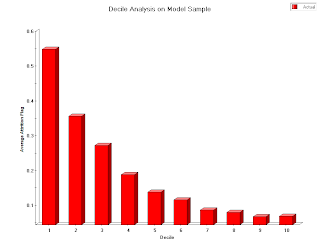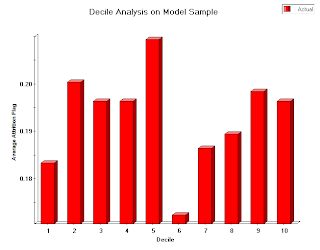# How to Interpret a Decile AnalysisAfter building a predictive model, there are several ways to determine how well the model is describing your data. One visual way to get an idea of how well a model is fitting your data is by taking a look at the decile analysis. Here we’ll take a look at what the decile analysis represents, how it’s created, and how to spot a good model.

## What a Decile Analysis Represents

After building a statistical model, a decile analysis is created to test the model’s ability to predict the intended outcome. Each column in the analysis chart represents a collection of records that have been scored using the model. The height of each column represents the average of those records’ actual behavior.

## How the Decile Analysis is Calculated

1. The hold-out or validation sample is scored according to the model being tested.
2. The records are sorted by their predicted scores in descending order and divided into ten equal-sized bins or deciles. The top decile contains 10% of the population most likely to respond and the bottom decile contains 10% of the population least likely to respond, based on the model scores.
3. The deciles and their actual response rates are graphed on the x and y axes, respectively.

After the decile analysis is built, you’ll want to take a look at the height of the bars in relation to one another. Deciding whether a model is worth moving forward with depends on the pattern you see when viewing the decile analysis.

## Ideal Situation: The Staircase Effect

When you’re looking at a decile analysis, you want to see a staircase effect;that is, you’ll want the bars to descend in order from left to right, as shown below.This is telling you that the model is “binning” your constituents correctly from most likely to respond to least likely to respond. A model exhibiting a good staircase decile analysis is one you can consider moving forward with.

## Not-So-Ideal Situations

In contrast, if the bars seem to be out of order (as shown below), the analysis is telling you that the model is not doing a very good job of predicting actual responses.If the bars seem to be the same height, or the decile analysis looks “flat”, it’s telling you that the model isn’t performing any better than randomly binning people into deciles would. In both cases, your model should be improved before moving forward with it.

## Stay up to dateSubscribe to our blog

Subscribe
Notify of